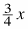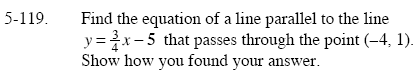### Home > GC > Chapter 5 > Lesson 5.3.5 > Problem5-119

5-119.

Find the equation of a line parallel to the line y =− 5 that passes through the point (−4, 1). Show how you found your answer. Homework Help ✎Since parallel lines have the same slope, the equation for the parallel line is:

$y=\frac{3}{4}x+b$

Since the new line goes through (−4,1), substitute x = −4 and y = 1 into the above equation, and then solve for b.

$y=\frac{3}{4}x+4$